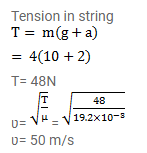# A 4.0 kg block is suspended from the ceiling of an elevator throughQuestion:

A $4.0 \mathrm{~kg}$ block is suspended from the ceiling of an elevator through a string having a linear mass density of $19.2 \times 10^{-3} \mathrm{~kg} / \mathrm{m}$. Find the speed (with respect to the string) with which a wave pulse can proceed on the string if the elevator accelerates up at the rate of $2.0 \mathrm{~m} / \mathrm{s}^{2}$.

Solution: